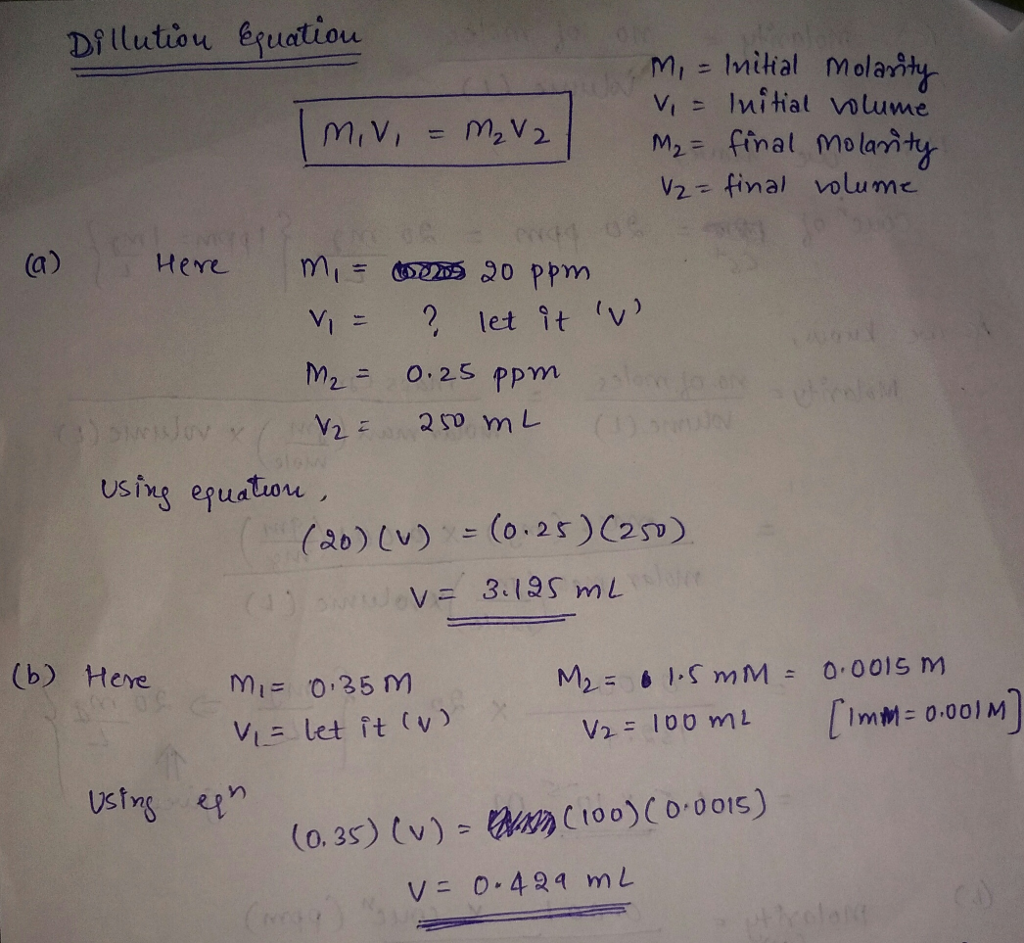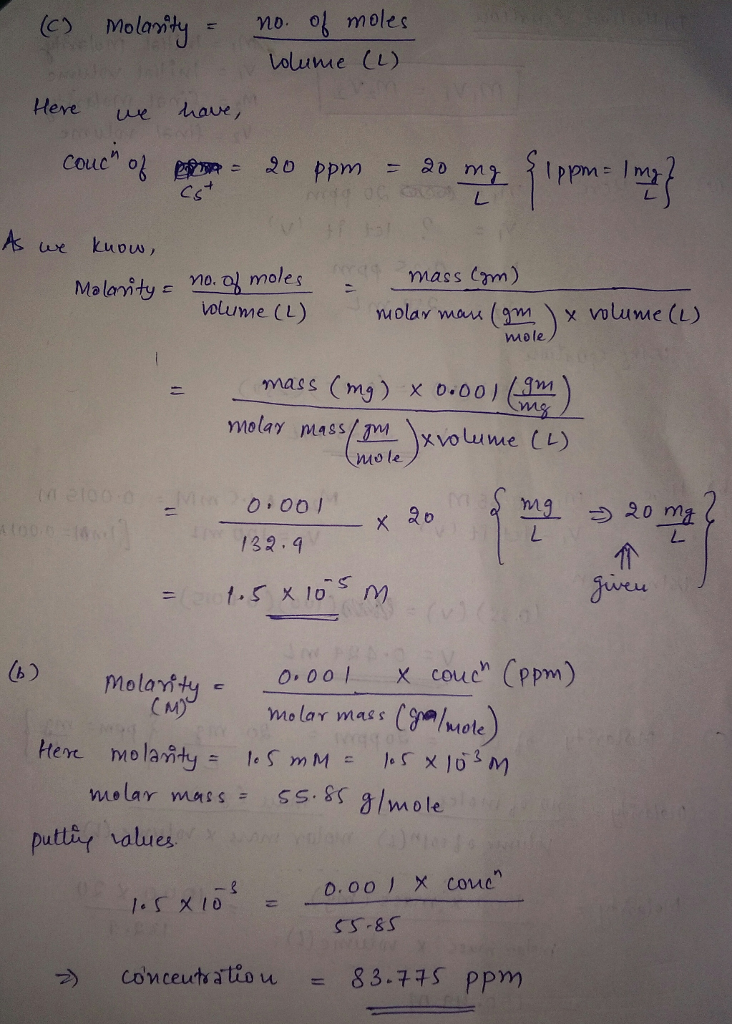# Question & Answer: Dilution Calculations a) How much of a 20 ppm solution of Cs' is needed to make a solution of…..

Dilution Calculations a) How much of a 20 ppm solution of Cs’ is needed to make a solution of o.25 ppm Cs’ in a 250 ml volumetric flask? b) How much of a 0.35 M solution of Fe2 is needed to make a solution of 1.5 mM Fe2 in a 100 ml volumetric flask? Conversion Calculations a) what is the molarity of a 20 ppm aqueous solution of Cs+ (Atomic weight Cs 132.9 g/mole)? b) What is the concentration in ppm of an